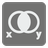Boolean Algebra
Simple
English DE EL ES FR IT JA RU UK

In mathematics and mathematical logic, Boolean algebra is a sub-area of algebra in which the values of the variables are true or false, typically denoted with 1 or 0 respectively. Instead of elementary algebra where the values of the variables are numbers and the main operations are addition and multiplication, the main operations of Boolean algebra are the conjunction (denoted ∧), the disjunction (denoted ∨) and the negation (denoted ¬).

In this mission you should implement some boolean operations:
- "conjunction" denoted x ∧ y, satisfies x ∧ y = 1 if x = y = 1 and x ∧ y = 0 otherwise.
- "disjunction" denoted x ∨ y, satisfies x ∨ y = 0 if x = y = 0 and x ∨ y = 1 otherwise.
- "implication" (material implication) denoted x→y and can be described as ¬ x ∨ y. If x is true then the value of x → y is taken to be that of y. But if x is false then the value of y can be ignored; however the operation must return some truth value and there are only two choices, so the return value is the one that entails less, namely true.
- "exclusive" (exclusive or) denoted x ⊕ y and can be described as (x ∨ y)∧ ¬ (x ∧ y). It excludes the possibility of both x and y. Defined in terms of arithmetic it is addition mod 2 where 1 + 1 = 0.
- "equivalence" denoted x ≡ y and can be described as ¬ (x ⊕ y). It's true just when x and y have the same value.

Here you can see the truth table for these operations:

``` x | y | x∧y | x∨y | x→y | x⊕y | x≡y |
--------------------------------------
0 | 0 |  0  |  0  |  1  |  0  |  1  |
1 | 0 |  0  |  1  |  0  |  1  |  0  |
0 | 1 |  0  |  1  |  1  |  1  |  0  |
1 | 1 |  1  |  1  |  1  |  0  |  1  |
--------------------------------------
```

You are given two boolean values x and y as 1 or 0 and you are given an operation name as described earlier. You should calculate the value and return it as 1 or 0.

Input: Three arguments. X and Y as 0 or 1. An operation name as a string.

Output: The result as 1 or 0.

Example:

`boolean(1,...`
You should be an authorized user in order to see the full description and start solving this mission.